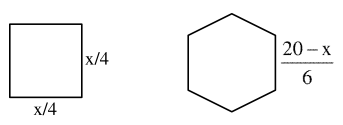# A wire of length

Question:

A wire of length $20 \mathrm{~m}$ is to be cut into two pieces. One of the pieces is to be made into a square and the other into a regular hexagon. Then the length of the side (in meters) of the hexagon, so that the combined area of the square and the hexagon is minimum, is:

1. $\frac{5}{2+\sqrt{3}}$

2. $\frac{10}{2+3 \sqrt{3}}$

3. $\frac{5}{3+\sqrt{3}}$

4. $\frac{10}{3+2 \sqrt{3}}$

Correct Option: , 4

Solution:

Let the wire is cut into two pieces of length $x$ and $20-x$.Area of square $=\left(\frac{x}{4}\right)^{2}$ Area of regular hexagon

$=6 \times \frac{\sqrt{3}}{4}\left(\frac{20-x}{6}\right)^{2}$

Total area $=\mathrm{A}(\mathrm{x})=\frac{\mathrm{x}^{2}}{16}+\frac{3 \sqrt{3}}{2} \frac{(20-\mathrm{x})^{2}}{36}$

$A^{\prime}(x)=\frac{2 x}{16}+\frac{3 \sqrt{3} \times 2}{2 \times 36}(20-x)(-1)$

$\mathrm{A}^{\prime}(\mathrm{x})=0$ at $\mathrm{x}=\frac{40 \sqrt{3}}{3+2 \sqrt{3}}$

Length of side of regular Hexagon $=\frac{1}{6}(20-x)$

$=\frac{1}{6}\left(20-\frac{4 \cdot \sqrt{3}}{3+2 \sqrt{3}}\right)$

$=\frac{10}{2+2 \sqrt{3}}$